# 问题三十一至四十

## 问题三十一：仿射变换（Afine Transformations）——倾斜

1. 使用仿射变换，输出（1）那样的$x$轴倾斜$30$度的图像（$t_x=30$），这种变换被称为X-sharing。
2. 使用仿射变换，输出（2）那样的y轴倾斜$30$度的图像（$t_y=30$），这种变换被称为Y-sharing。
3. 使用仿射变换，输出（3）那样的$x$轴、$y$轴都倾斜$30$度的图像($t_x = 30$，$t_y = 30$)。void leanAt(Mat& src, double x_sharing, double y_sharing) {
Mat result = Mat::zeros(src.rows, src.cols, CV_8UC3);
result.forEach<Vec3b>([&](Vec3b& pix, const int * position) {
int x = position;
int y = position;
int cur_pos = { x,y };
Mat m(Size(1, 2), CV_32SC1, cur_pos);
double a_x = x_sharing / src.rows;
double a_y = y_sharing / src.cols;
Mat ori_pos = AfineTransformations(m, 1,a_x,a_y,1, 0, 0);
//printMat(ori_pos);
for (int i = 0; i < 3; i++) {
int ori_pos_x = ori_pos.at<Vec<double, 1>>(0, 0);
int ori_pos_y = ori_pos.at<Vec<double, 1>>(1, 0);
if (ori_pos_x >= src.rows || ori_pos_y >= src.cols || ori_pos_x < 0 || ori_pos_y < 0) {
pix[i] = 0;
}
else
pix[i] = src.at<Vec3b>(ori_pos_x, ori_pos_y)[i];
}
});
src = result;
}

### Show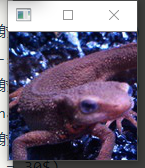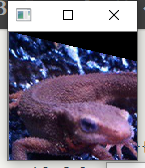### Note

• 利用OpenCV的API进行仿射变换

• 第一步：获取仿射映射矩阵（两种）

getAffineTransform()       //三点法
Mat M1=getAffineTransform(const Point2f* src, const Point2f* dst)
//参数const Point2f* src：原图的三个固定顶点

//参数const Point2f dst：目标图像的三个固定顶点
//返回值：Mat型变换矩阵，可直接用于warpAffine()函数
//注意，顶点数组长度超过3个，则会自动以前3个为变换顶点；数组可用Point2f[]或Point2f
表示


cpp
getRotationMatrix2D       //直接指定比例和角度
Mat M2=getRotationMatrix2D (CvPoint2D32f  center,double angle,double scale)
//参数CvPoint2D32f  center，表示源图像旋转中心
//参数double angle，旋转角度，正值表示逆时针旋转
//参数double scale，缩放系数
• 第二步：进行仿射变换
C++ void warpAffine(InputArray src, OutputArray dst, InputArray M, Size dsize, int flags=INTER_LINEAR, int borderMode=BORDER_CONSTANT, const Scalar& borderValue=Scalar())
//参数InputArray src：输入变换前图像
//参数OutputArray dst：输出变换后图像，需要初始化一个空矩阵用来保存结果，不用设定矩阵尺寸
//参数InputArray M：变换矩阵，用另一个函数getAffineTransform()计算
//参数Size dsize：设置输出图像大小
//参数int flags = INTER_LINEAR：设置插值方式，默认方式为线性插值(另一种WARP_FILL_OUTLIERS)
//参数int borderMode=BORDER_CONSTANT：边界像素模式，默认值BORDER_CONSTANT
//参数const Scalar& borderValue=Scalar()，在恒定边界情况下取的值，默认值为Scalar（），即0
第五个参数插值方式可选方式： 标识符 含义
INTER_NEAREST 最邻近插值
INTER_LINEAR 线性插值(默认)
INTER_CUBIC 三次样条插值
INTER_AREA 区域插值
INTER_LANCZOS4 LancZoc4 插值
INTER_LINEAR_EXACT 双线性插值
INTER_MAX 插补码掩码
WARP_FILL_OUTLIERS 填充所有目标图像像素。如果它们中的一些对应于源图像，它们被设置为0
WARP_INVERSE_MAP 逆变换

## 问题三十二：傅立叶变换（Fourier Transform）

$$G(k,l)=\frac{1}{H\ W}\ \sum\limits{y=0}^{H-1}\ \sum\limits{x=0}^{W-1}\ I(x,y)\ e^{-2\ \pi\ i\ (\frac{k\ x}{W}+\frac{l\ y}{H})}$$

$$I(x,y)=\frac{1}{H\ W}\ \sum\limits{l=0}^{H-1}\ \sum\limits{k=0}^{W-1}\ G(l,k)\ e^{2\ \pi\ i\ (\frac{k\ x}{W}+\frac{l\ y}{H})}$$

### Principe

傅里叶变换可以将一副图片分解为正弦和余弦两个分量，换言之，它可以将一幅图像从空间域（spatial domain）转换为频域（frequency domain）。这种变换的思想是任何函数可以很精确的接近无穷个sin()函数和cos()函数的和。

• 空间域
一般情况下，空间域的图像是f(x, y) = 灰度级（0-255），形象一点就是一个二维矩阵，每一个坐标对应一个颜色值
• 频率域
对于一个正弦信号，如果它的幅度变化很快，我们称之为高频信号，如果变化非常慢，我们称之为低频信号。迁移到图像中，图像哪里的幅度变化非常大呢？边界点或者噪声。所以我们说边界点和噪声是图像中的高频分量（这里的高频是指变化非常快，不是出现的次数多），图像的主要部分集中在低频分量。

$$F(k,l)= \sum\limits{y=0}^{N-1}\ \sum\limits{x=0}^{N-1}\ f(x,y)\ e^{-2\ \pi\ j\ (\frac{k\ x}{N}+\frac{l\ y}{N})}$$
$$e^{ix}=cos x+i sin x$$

toGray()函数在之第一章声明过,也可是使用OpenCV自带的API来转换灰度图
• 傅里叶变换

//// Discrete Fourier transformation
//这个奇怪的vector就是用来存转换后的频域的
std::vector<
std::vector<
std::complex<double>>> DFT(Mat& src) {
int width = src.cols;
int height = src.rows;
std::complex<double> val;
Mat grey = toGray(src);
std::vector<std::vector<std::complex<double>>> FTMap;
Mat result = Mat::zeros(width, height, CV_8UC1);

//j,k用来表示频谱图中的坐标
for (int j = 0; j < width; j++) {
std::vector<std::complex<double>> MapLine;
for (int k = 0; k < height; k++) {
val.real(0);
val.imag(0);

//x,y表示时域中的坐标
for (int x = 0; x < width; x++) {
for (int y = 0; y < height; y++) {
uchar I = grey.at<Vec<uchar, 1>> (x, y);
double theta = (double)-2 * 3.1415926*(((double)k*(double)y / (double)height) + ((double)j*(double)x / (double)width));
val += (double)I*std::complex<double>(cos(theta), sin(theta));
}
}
val /= sqrt((double)width*(double)height);
result.at<Vec<uchar, 1>>(j, k) = (uchar)sqrt(val.imag()*val.imag() + val.real()*val.real());
MapLine.push_back(val);
}
FTMap.push_back(MapLine);
}
//输出以下现在是什么样的图案
imshow("FDT", result);
return FTMap;
}
• 傅里叶逆变换

Mat IDFT(std::vector<std::vector<std::complex<double>>> FTMap) {
Mat result = Mat::zeros(128, 128, CV_8UC1);
int width=128, height = 128;

std::complex<double> val;

for (int j = 0; j < width; j++) {
std::vector<std::complex<double>> MapLine;
for (int k = 0; k < height; k++) {
val.real(0);
val.imag(0);

//x,y表示时域中的坐标
for (int x = 0; x < width; x++) {
for (int y = 0; y < height; y++) {
std::complex<double> G = FTMap[x][y];
double theta = (double)2 * 3.1415926*(((double)k*(double)y / (double)height) + ((double)j*(double)x / (double)width));
val += G*std::complex<double>(cos(theta), sin(theta));
}
}
result.at<Vec<uchar, 1>>(j, k) = (uchar)(std::abs(val) / sqrt(height * width));
}
}
return result;
• 在main函数中这样调用就可以了

Mat img = imread("G:\\cv\\ImageProcessing100Wen-master\\Question_21_30\\imori.jpg");
imshow("原图", img);
imshow("effect",IDFT(DFT(img)));

### SHow

• 原图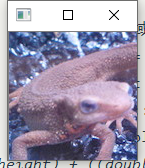• 转换后的频域图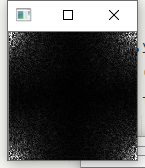• 逆变换的复原图### Note

• 可以看到这种方法来求傅里叶变换以及傅里叶逆变换的时间复杂度非常高,达到$O(n^4)$,所以常用的方法是FFT,ji快速傅里叶变换,在之后的题目中会介绍到

## 问题三十三：傅立叶变换——低通滤波

imori.jpg灰度化之后进行傅立叶变换并进行低通滤波，之后再用傅立叶逆变换复原吧！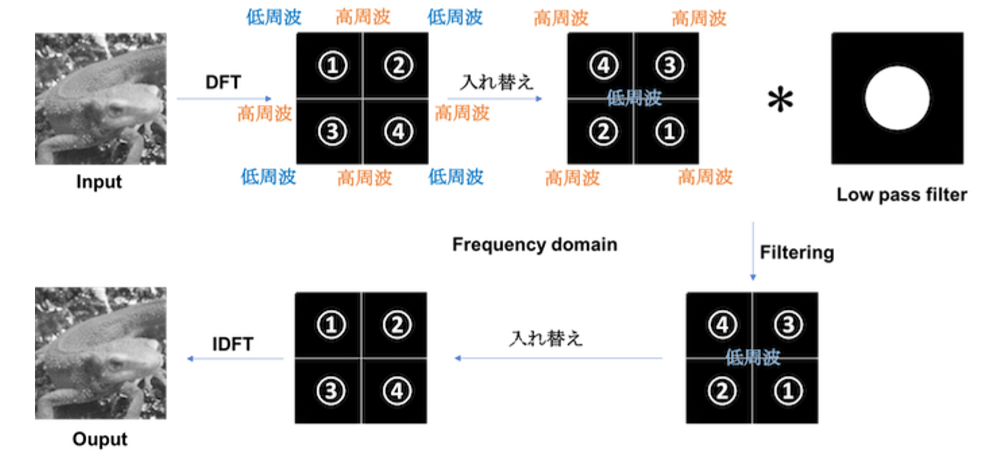//低频滤波
Mat LowPassFilter(Mat& src, double pass_r) {
auto map = DFT(src);
int width = src.cols;
int height = src.rows;
for (int i = 0; i < width/2; i++) {
for (int j = 0; j < height/2; j++) {
if (sqrt(i * i + j * j) >= pass_r * height / 2) {
map[i][j] = 0;
map[width - i-1][j] = 0;
map[i][height - j-1] = 0;
map[width - i - 1][height - j - 1] = 0;
}
}
}
return IDFT(map);
}

### Show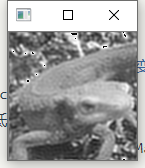## 问题三十四：傅立叶变换——高通滤波

imori.jpg灰度化之后进行傅立叶变换并进行高通滤波，之后再用傅立叶逆变换复原吧！

//高通滤波
Mat HighPassFilter(Mat& src, double pass_r) {
auto map = DFT(src);
int width = src.cols;
int height = src.rows;
for (int i = 0; i < width / 2; i++) {
for (int j = 0; j < height / 2; j++) {
if (sqrt(i * i + j * j) <= pass_r * height / 2) {
map[i][j] = 0;
map[width - i - 1][j] = 0;
map[i][height - j - 1] = 0;
map[width - i - 1][height - j - 1] = 0;
}
}
}
return IDFT(map);
}

### Show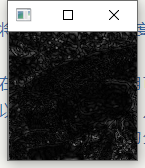## 问题三十五：傅立叶变换——带通滤波

imori.jpg灰度化之后进行傅立叶变换并进行带通滤波，之后再用傅立叶逆变换复原吧！

//带通滤波
Mat BandPassFilter(Mat& src, double pass_start, double pass_end) {
auto map = DFT(src);
int width = src.cols;
int height = src.rows;
for (int i = 0; i < width / 2; i++) {
for (int j = 0; j < height / 2; j++) {
if (sqrt(i * i + j * j) <= pass_start * height / 2|| sqrt(i * i + j * j)>= pass_end * height / 2) {
map[i][j] = 0;
map[width - i - 1][j] = 0;
map[i][height - j - 1] = 0;
map[width - i - 1][height - j - 1] = 0;
}
}
}
return IDFT(map);
}

### Show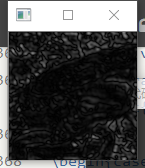## 问题三十六：JPEG 压缩——第一步：离散余弦变换（Discrete Cosine Transformation）

imori.jpg`灰度化之后，先进行离散余弦变换，再进行离散余弦逆变换吧！ここでは画像を8x8ずつの領域に分割して、各領域で以上のDCT, IDCTを繰り返すことで、JPEG符号に応用される。 今回も同様に8x8の領域に分割して、DCT, IDCTを行え。

——gzr

## 问题三十七：PSNR

$$\text{PSNR}=10\ \log{10}\ \frac{{v{max}}^2}{\text{MSE}}\ \text{MSE}=\frac{\sum\limits{y=0}^{H-1}\ \sum\limits{x=0}^{W-1}\ [I_1(x,y)-I_2(x,y)]^2}{H\ W}$$

$$\text{bit rate}=8\ \frac{K^2}{8^2}$$

1. 这里应该有个公式的，但是它不知道去哪儿了。

2. 下面图里文字的意思：高周波=高频；低周波=低频；入れ替え=替换。

## 发布者